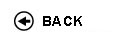Politecnico di Torino
Academic Year 2017/18
04KWQLI, 04KWQJM, 04KWQLM, 04KWQLN, 04KWQLP, 04KWQLS, 04KWQLX, 04KWQLZ, 04KWQMA, 04KWQMB, 04KWQMC, 04KWQMH, 04KWQMK, 04KWQMN, 04KWQMO, 04KWQMQ, 04KWQNX, 04KWQOA, 04KWQOD, 04KWQPC, 04KWQPI, 04KWQPL
Mathematical analysis I
1st degree and Bachelor-level of the Bologna process in Automotive Engineering - Torino
1st degree and Bachelor-level of the Bologna process in Mechanical Engineering - Torino
1st degree and Bachelor-level of the Bologna process in Computer Engineering - Torino
Espandi...
 Teacher Status SSD Les Ex Lab Tut Years teaching Adami Riccardo O2 MAT/05 60 40 0 0 3 Mercadante Silvio 60 40 0 0 2
 SSD CFU Activities Area context MAT/05 10 A - Di base Matematica, informatica e statistica
 Subject fundamentals This course represents a bridge between high school and university. The main goal of the course is to provide the students with tools to follow the concatenation of simple logical arguments, and to introduce the fundamentals of differential and integral calculus for functions of one variable, with applications to ordinary differential equations of the first and the second order. Expected learning outcomes Ability to follow a chain of logical arguments. Knowledge of the fundamentals of differential and integral calculus for functions of one variable. Gaining an adequate ability in computation. Prerequisites / Assumed knowledge Numerical sets, equations and inequalities, analytic geometry and trigonometry. Elementary functions and their basic properties. Contents Preliminaries: sets, operations with sets and logical symbols. Numerical sets, maxima, minima and extrema. The completeness property of real numbers and its consequences. Functions: surjectivity and injectivity; composition of functions, inverse functions. Functions of one real variable: elementary functions, monotone functions and inverse functions. (About 15 hours) Limits and continuity: limits of functions and sequences; continuity. Theorems on limits: uniqueness of the limit, sign-preserving property and local boundedness, comparison theorems. Limits of monotone functions. Algebra of limits. Indeterminate forms. Local comparison of functions. Landau symbols. Infinite and infinitesimal functions. Order of an infinity and of an infinitesimal, principal part with respect to a test function. Asymptotes. The number e. Fundamental trigonometric and exponential limits. Functions continuous over an interval: existence of zeros and of maxima and minima. (About 24 hours) Derivatives: geometrical and physical meaning. Computation of derivatives. Derivatives of elementary functions. Derivatives and continuity. Non-differentiability points, extremal and critical points. Fermat Theorem. Functions differentiable over an interval and fundamental theorems of differential calculus (Rolle and Lagrange) and their consequences. De L'H�pital rule. Taylor formula and fundamental Maclaurin expansions. Use of Taylor expansions in the local analysis of functions: comparison, extrema and convexity. Applications to the study of the graph of a function. (About 23 hours) Primitives and their computation; primitives of rational functions. Indefinite integral. The definite integral of a piecewise continuous function. Properties of the integral. Integral mean, the mean value theorem and the Fundamental Theorem of Calculus. Relations between definite integrals and primitives. Improper integrals: definitions and convergence tests. (About 21 hours) Complex numbers and differential equations: algebraic and trigonometric form of a complex number. Real and imaginary part, modulus and argument. Roots of complex numbers; the Fundamental Theorem of Algebra. The exponential of a complex number and the Euler formula. Ordinary differential equations: the Cauchy problem. First order ordinary differential equations, linear or with separable variables. Second order linear differential equations with constant coefficients. (About 17 hours) Delivery modes The course consists of 60 hours of lectures and 40 hours of exercise classes. Texts, readings, handouts and other learning resources C. Canuto, A. Tabacco. Mathematical Analysis I. Springer-Verlag UTX, 2015. Additional material will be provided by the teacher and will be available in the Internet personal page of the student. Assessment and grading criteria The main purpose of the exam is to ascertain both the theoretical knowledge of the subject and the ability to apply theory to the solution of exercises. It consists of a multiple-choice test and a written exam, possibly followed by an oral exam. The final mark is determined by the scores of the test and the written exam and, if the oral exam takes place, its result is also taken into account. TEST. This part of the exam, which lasts one hour and takes place in a computer lab, consists of 20 multiple-choice queries, including theoretical questions and covering all the syllabus. Books or other teaching material cannot be consulted. Each question is worth one point, and the maximum achievable score is 20. If the score is less than 12 then the exam is failed, otherwise the student proceeds with the written exam. WRITTEN EXAM. This part lasts 75 minute, and typically consists of two exercises. Of these, the former is the study of a function, while the latter is devoted to some more specific part of the syllabus and may include theoretical questions. Books or other teaching material cannot be consulted. The maximum achievable score is 13. If the score is less than 5 then the exam is failed, otherwise the final score of the exam is obtained as the sum of the scores of the test and the written part, unless an oral examination is required by the teacher (or by the student, provided that the final score is at least 18). ORAL EXAM. This part of the exam, which can be requested by the teacher or by the student as described above, mainly aims at ascertaining the theoretical knowledge of the subject, and is a further element of appraisal. In case this part of the exam takes place, its result will contribute (together with the scores of the test and the written exam) to the final exam score. Programma definitivo per l'A.A.2017/18© Politecnico di Torino
Corso Duca degli Abruzzi, 24 - 10129 Torino, ITALY